Get instant live expert help with Excel or Google Sheets“My Excelchat expert helped me in less than 20 minutes, saving me what would have been 5 hours of work!”

#### Post your problem and you’ll get expert help in seconds.

Your message must be at least 40 characters
Our professional experts are available now. Your privacy is guaranteed.

# How To Create Equations Using the Equation Editor

We can use the Equation editor to insert, write or format Equations. In this tutorial, we will learn how to write, insert, solve or format Equations using the Equation Editor effectively.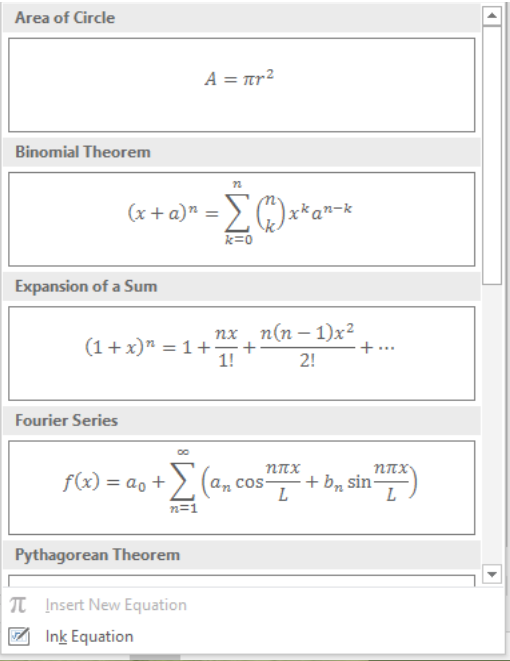Figure 1 – How to add equations

## How to Insert an Equation using the Equation Editor

• We will go to the Insert tab and navigate to the Symbols group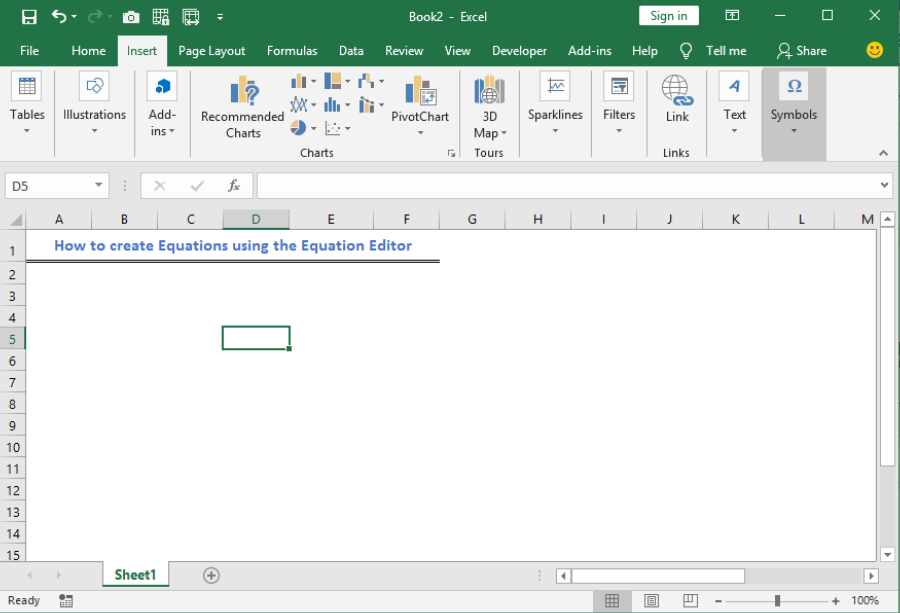Figure 2 – How to enter a formula

• We will click on the drop-down arrow to view the Equations and Symbols icons.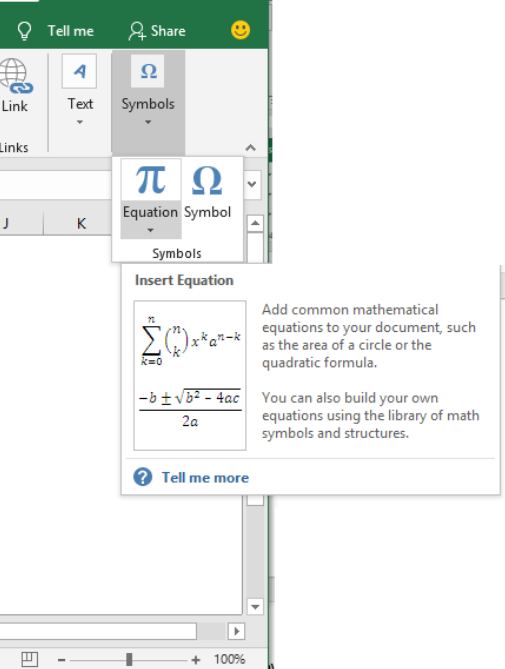Figure 3 – How to insert an equation

• Next, we will click again on the drop-down arrow to the side of the Equation arrow.
• This will show a list of preinstalled equations.  We will click on any of these Equations to insert it into our Excel sheet.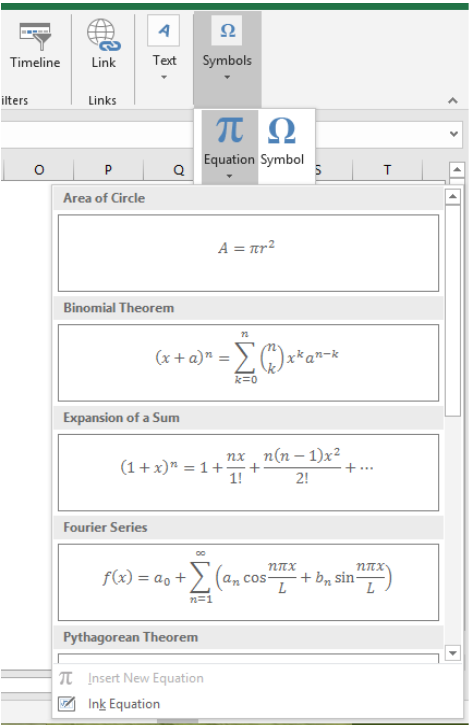Figure 4 – Enter formula

• Let us click on the Expansion of a Sum Equation.
• Now, we will select OK.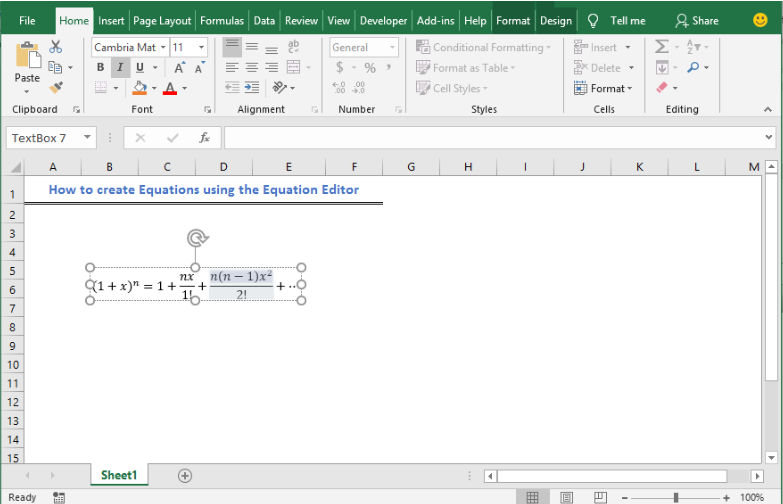Figure 5 – Insert Equation

## Write an equation using Equation Editor (How to input a formula)

• If we want to create a formula of our own, we will go to the Insert tab and navigate to the Symbols group
• Next, we will click on the Equation.
• This will input a text box“Type Equation here” in our Excel sheet.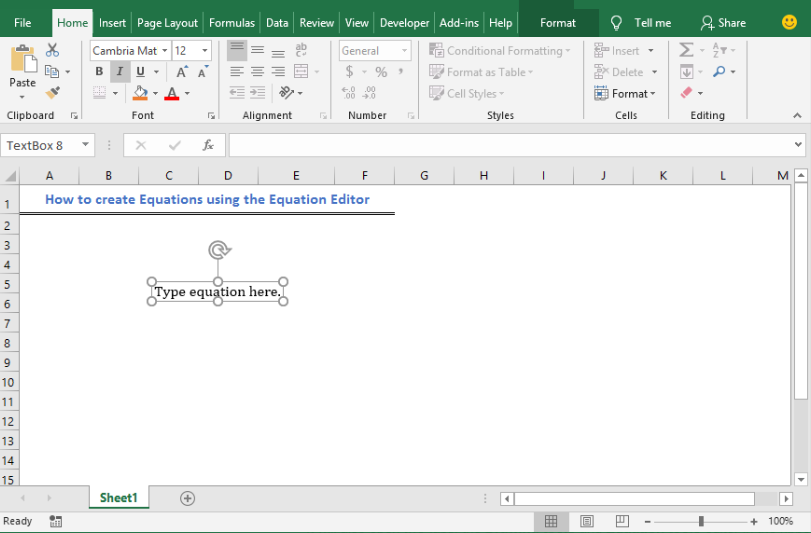Figure 6 – How to add equations

• It will also open two tabs, the Drawing tools, and Equation tools editor in the ribbon at the top of our worksheet.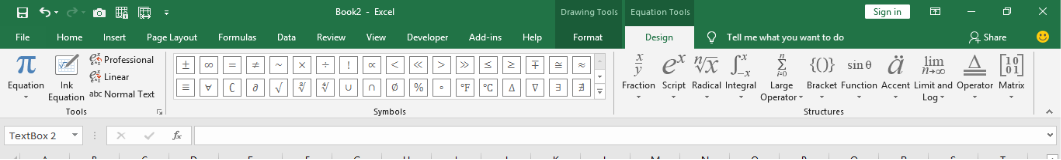Figure 7 – Write or Insert an equation

• We can use the preinstalled Equations by clicking the drop-down arrow under the Equations icon.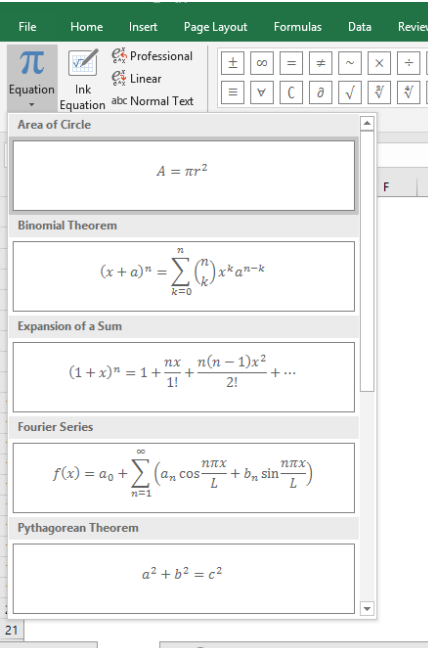Figure 8 – Enter formula

• We can equally create our own Equations by using the Symbols tab alongside other kinds of functions we find in the Equations tools tab.
• We can use a wide range of other operations in the Symbols group.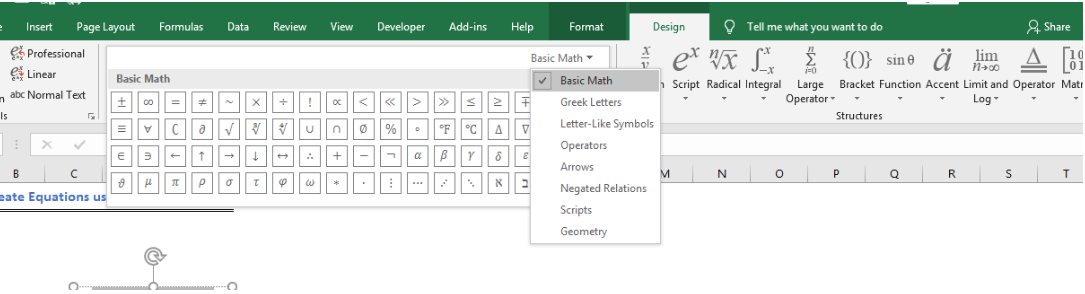Figure 9 – Insert Equation Tab

• The Structures group gives us different ways to insert particular things. For example, if we want to enter a fraction in our Excel sheet. We can select any of the fractions options.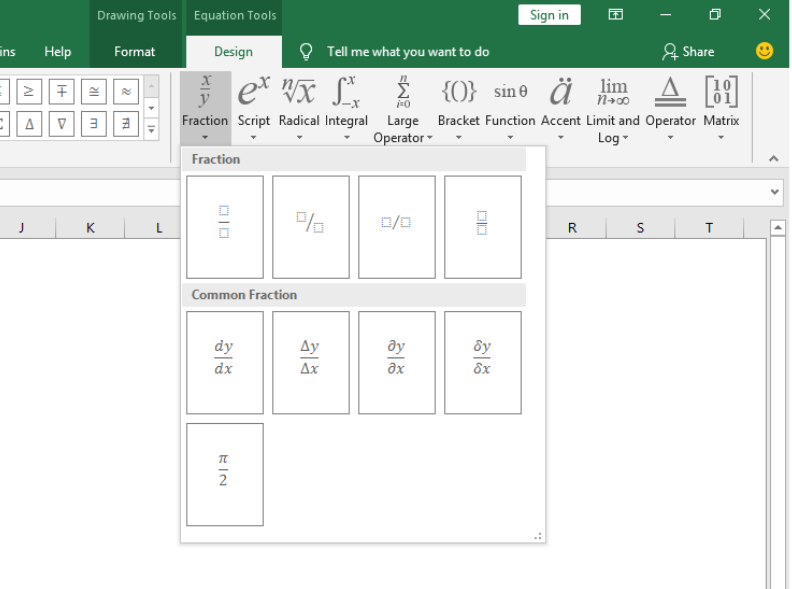Figure 10 – How to add equations using definite structures.

• Next, we will enter our values into the pop-up boxes in our Excel sheet.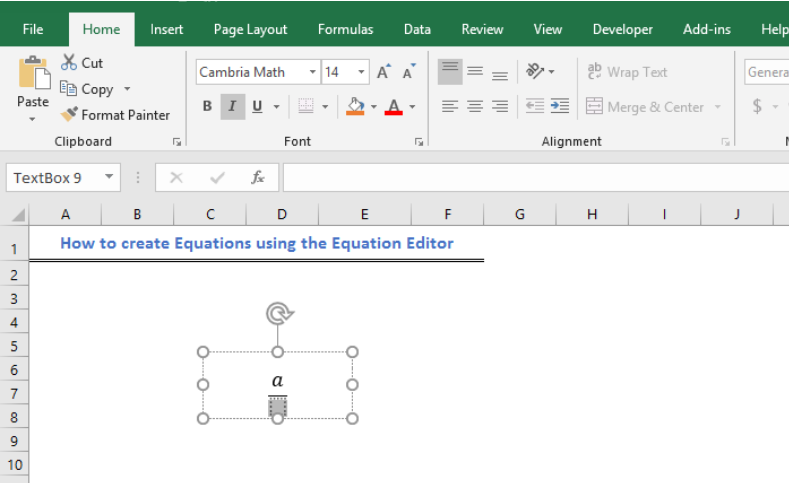Figure 11 – How to input a formula

## Formatting our Equations

• We can always resize our Equations by right-clicking and Increasing font size.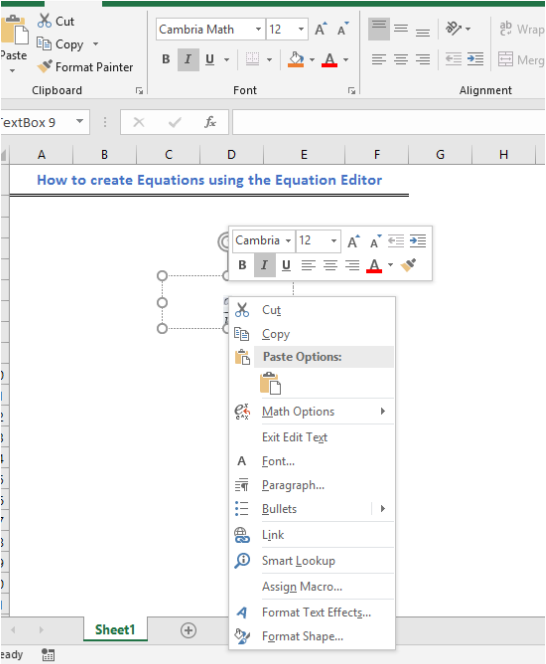Figure 12 – Format formula using Equations editor

• In addition, we can embed formula into a cell. This means we can make our formula stay within a cell rather than move around as a floating object. To do this:
• We will right-click on the Text box and select Format Shape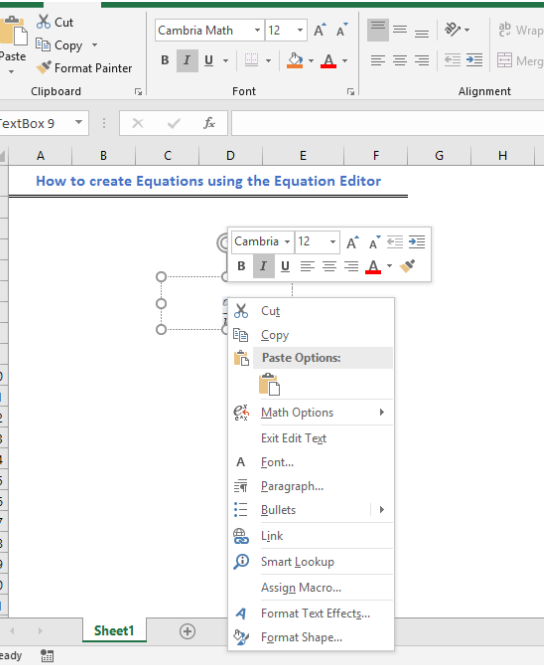Figure 13 – How to format equation

• Next, we will select Size and Properties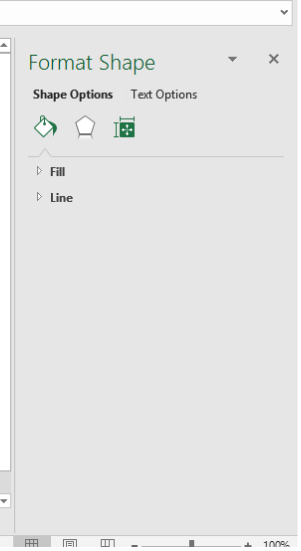Figure 14 – Format Shape using Size and Properties.

• And lastly, we will mark “Move and size with cells”.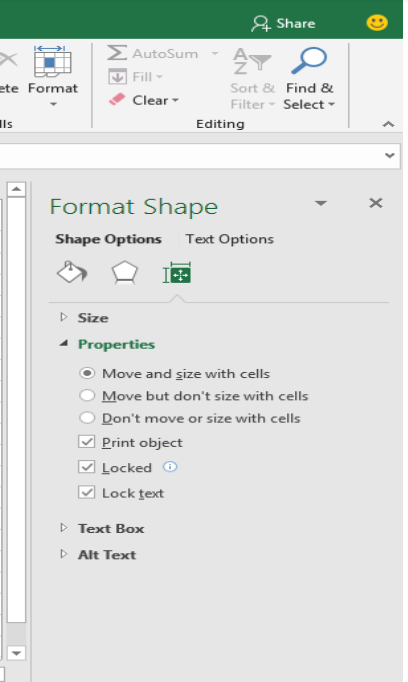Figure 15 – Format to Move and size with cells

## Instant Connection to an Excel Expert

Most of the time, the problem you will need to solve will be more complex than a simple application of a formula or function. If you want to save hours of research and frustration, try our live Excelchat service! Our Excel Experts are available 24/7 to answer any Excel question you may have. We guarantee a connection within 30 seconds and a customized solution within 20 minutes.